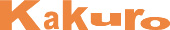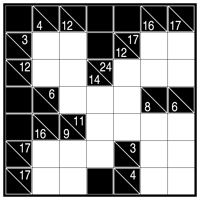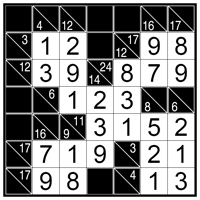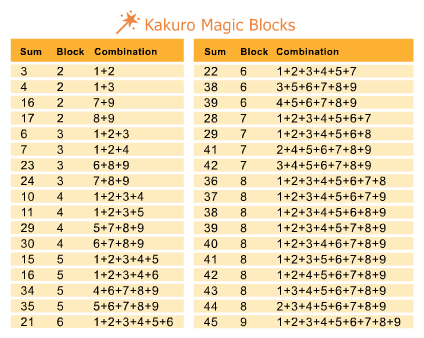﻿ Kakuro правила
 ВходРегистрация# Kakuro правила

Kakuro are easy to learn yet highly addictive language-independent logic puzzles now following the footsteps of the worldwide Sudoku success. Requiring just pure logic and simple add/subtract calculations, these numerical-crossword puzzles will carry you into a fascinating world of number combinations you never imagined could exist.

Best defined as number crosswords, Kakuro puzzles come in endless variations, are available in almost any grid size and range from very easy to extremely difficult taking anything from ten minutes to several hours to solve. Make one mistake and you’ll find yourself stuck later on as you get closer to the solution...

If you like Sudoku and other logic puzzles, you will love Conceptis Kakuro as well!

## Classic Kakuro

Each puzzle consists of a blank grid with sum-clues in various places. The object is to fill all empty squares using numbers 1 to 9 so the sum of each horizontal block equals the clue on its left, and the sum of each vertical block equals the clue on its top. In addition, no number may be used in the same block more than once.## Holey Kakuro

Each puzzle consists of a blank grid with sum-clues in various places. The object is to fill all empty squares using numbers 1 to 9 so the sum of each horizontal block equals the clue on its left, and the sum of each vertical block equals the clue on its top. In addition, no number may be used in the same block more than once.

## Round Kakuro

Each puzzle consists of a blank grid with sum-clues in various places. The object is to fill all empty squares using numbers 1 to 9 so the sum of each horizontal block equals the clue on its left, and the sum of each vertical block equals the clue on its top. In addition, no number may be used in the same block more than once.

## Multi Kakuro

Each puzzle consists of a blank grid with sum-clues in various places. The object is to fill all empty squares using numbers 1 to 9 so the sum of each horizontal block equals the clue on its left, and the sum of each vertical block equals the clue on its top. In addition, no number may be used in the same block more than once.

## Diamond Kakuro

Each puzzle consists of a blank grid with sum-clues in various places. The object is to fill all empty squares using numbers 1 to 9 so the sum of each horizontal block equals the clue on its left, and the sum of each vertical block equals the clue on its top. In addition, no number may be used in the same block more than once.

## Heart Kakuro

Each puzzle consists of a blank grid with sum-clues in various places. The object is to fill all empty squares using numbers 1 to 9 so the sum of each horizontal block equals the clue on its left, and the sum of each vertical block equals the clue on its top. In addition, no number may be used in the same block more than once.

## Kakuro Magic Blocks

The secret to solving Kakuro puzzles is learning how to use magic blocks – those special situations where only a single combination of numbers can fit into a block of a given length. For example, if 6 is the sum-clue of a block of three squares then the block must consist of the numbers 1+2+3 but not necessarily in this order.When spotting a magic block, the Magic Blocks table can be helpful to identify which unique numbers must be used in that block. The only thing left is to find out in which order the numbers should be organized.

This Kakuro Magic Blocks table shows all the magic block possibilities which may occur in Kakuro puzzles.